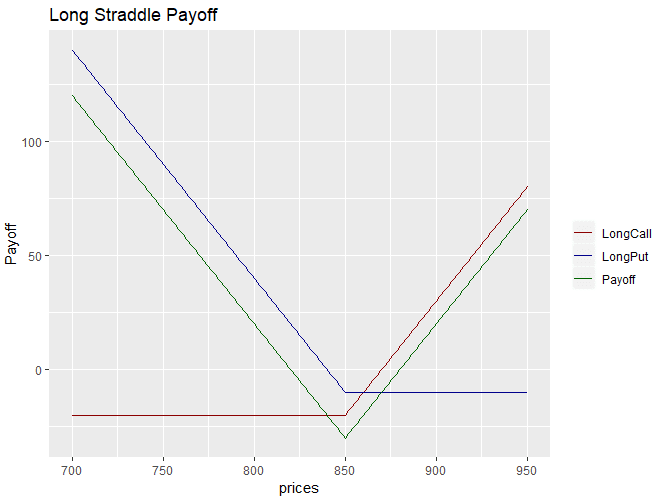# Options Strategy: Create Long Straddle with R Language

The Long Straddle is an options trading strategy that involves going long on a call option and a put option with the same underlying asset, same expiration and same strike price. This strategy tries to gain profits due to volatility in either direction as the strategy wins when the price movement is significant in any direction. In this article we will learn about how to construct a long straddle with R programming language.

The code for implementing the long straddle with R is presented below. We first create a vector of prices using the seq() function in R. For our example, we create a vector with prices ranging from 700 to 950. We chose the strike price to be 850. The call and put options have a premium of 20 and 10 respectively. We calculate the payoffs from the call and the put options and then calculate the payoff from the long straddle strategy with a long position in both call and the put option. We finally plot the strategy payoff using the ggplot library in R.

prices <- seq(700,950,1) # Vector of prices
strike <- 850 # strike price for both put and call
premium_call <- 20 # option price call
premium_put <- 10  # option price put

# call option payoff at expiration
intrinsicValuesCall <- prices - strike - premium_call

# put option payoff at expiration
intrinsicValuesPut <- strike - prices - premium_put

# The payoff of the Strategy is the sum of the call and put payoff. Need
# to sum wise element by element between the two vectors
payoff <- rowSums(cbind(payoffLongCall,payoffLongPut))

# Make a DataFrame with all the variable to plot it with ggplot
results <- data.frame(cbind(prices,payoffLongCall,payoffLongPut,payoff))

ggplot(results, aes(x=prices)) +
geom_line(aes(y = payoffLongCall, color = "LongCall")) +
geom_line(aes(y = payoffLongPut, color="LongPut"))+
geom_line(aes(y=payoff, color = 'Payoff')) +
scale_colour_manual("",
breaks = c("LongCall", "LongPut", "Payoff"),
values = c("darkred", "darkblue", "darkgreen")) + ylab("Payoff")+